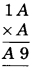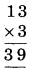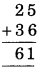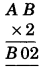# CBSEtips.in

## Wednesday 24 February 2021

### CBSE Class 8 Maths - MCQ and Online Tests - Unit 16 - Playing with Numbers

#### CBSE Class 8 Maths – MCQ and Online Tests –  Unit 16 – Playing with Numbers

Every year CBSE students attend Annual Assessment exams for 6,7,8,9,11th standards. These exams are very competitive to all the students. So our website provides online tests for all the 6,7,8,9,11th standards’ subjects. These tests are also very effective and useful for those who preparing for any competitive exams like Olympiad etc. It can boost their preparation level and confidence level by attempting these chapter wise online tests.

These online tests are based on latest CBSE syllabus. While attempting these, our students can identify their weak lessons and continuously practice those lessons for attaining high marks. It also helps to revise the NCERT textbooks thoroughly

#### CBSE Class 8 Maths – MCQ and Online Tests – Unit 16 – Playing with Numbers

Question 1.
Which of the following numbers is not divisible by 3 ?
(a) 234
(b) 243
(c) 324
(d) 457.

Hint:
4 + 5 + 7 = 16 is not divisible by 3.

Question 2.
If the three digit number 24x is divisible by 9, the value of x is:
(a) 3
(b) 7
(c) 1
(d) None of the above

Hint:
If 24x is divisible by 9, then:
2 + 4 + x = 6 + x is also divisible by 9.
It is possible only when, 6 + x = 9, 18, Ö
Since x is single digit, therefore, 6 + 3 = 9
x = 3

Question 3.
Which of the following numbers is divisible by 3 ?
(a) 145
(b) 237
(c) 709
(d) 400.

Hint:
2 + 3 + 7 = 12 is divisible by 3.

Question 4.
The number 100 ◊ b + 10 ◊ c + a in usual form is
(a) bac
(b) bca
(c) cab
(d) cba.

Question 5.
Which of the following numbers is divisible by 9 ?
(a) 234
(b) 334
(c) 444
(d) 434.

Hint:
2 + 3 + 4 = 9 is divisible by 9.

Question 6.
The number 10 ◊ 2 + 7 in usual form is
(a) 72
(b) 22
(c) 77
(d) 21.

Question 7.
The generalised form of the number 123 is
(?) 1 ◊ 100 + 2 ◊ 10 + 3
(b) 2 ◊ 100 + 3 ◊ 10 + 1
(c) 3 ◊ 100 + 1 ◊ 10 + 2
(d) none of these.

Answer: (?) 1 ◊ 100 + 2 ◊ 10 + 3

Question 8.
The generalised form of the number 234 is
(?) 2 ◊ 100 + 3 ◊ 10 + 4
(b) 3 ◊ 100 + 4 ◊ 10 + 2
(c) 4 ◊ 100 + 2 ◊ 10 + 3
(d) none of these.

Answer: (?) 2 ◊ 100 + 3 ◊ 10 + 4

Question 9.
The number 3 ◊ 100 + 4 ◊ 10 + 5 in usual form is
(a) 453
(b) 435
(c) 354
(d) 345.

Question 10.
The number 5 ◊ 100 + 7 ◊ 10 + 9 in usual form is
(a) 795
(b) 759
(c) 579
(d) 597.

Question 11.
The number 100 ◊ a + 10 ◊ 6 + c in usual form is
(a) abc
(b) bca
(c) cab
(d) none of these.

Question 12.
Find the value of A in the following:(a) 1
(b) 2
(c) 3
(d) 4.

Hint:Question 13.
Find the value of A, B in the following:(a) 5, 6
(b) 5, 5
(c) 6, 5
(d) 6, 6.

Hint:Question 14.
Find the value of A, B in the following:(a) 3, 1
(b) 1, 1
(c) 3, 3
(d) 1, 3.

Hint:Question 15.
Find the value of A, B in the following:(a) 6, 9
(b) 6, 6
(c) 9, 6
(d) 9, 9

Hint:Question 16.
Find the values of A, B in the following:(a) 5, 1
(b) 5, 5
(c) 1, 1
(d) 1, 5.

Hint:Question 17.
Which of the following numbers is divisible by 2 ?
(a) 19
(b) 27
(c) 99
(d) 50.

Hint:
In 19, 27, 99, oneís digit is not divisible by 2.

Question 18.
Which of the following numbers is divisible by 2 ?
(a) 179
(b) 235
(c) 500
(d) 673.

Hint:
In 500, oneís digit is divisible by 2.

Question 19.
If the number 1 x 8 is divisible by 3, then x is equal to
(a) 0 or 3 or 6 or 9
(b) 4
(c) 5
(d) 7.

Answer: (a) 0 or 3 or 6 or 9
Hint:
1 + 0 + 8 = 9
1 + 3 + 8 = 12;
9, 12, 15, 18 each is divisible by 3

Question 20.
If the number 9 y 7 is a multiple of 3, then y =
(a) 4
(b) 3
(c) 6
(d) 2 or 5 or 8.

Answer: (d) 2 or 5 or 8.
Hint:
9 + 2 + 7 = 18
9 + 5 + 7 = 21;
9 + 8 + 7 = 24.
each of 18, 21 and 24 is divisible by 3.

Question 21.
If the three-digit number 4 3 x is divisible by 9, what is the value of x ?
(a) 1
(b) 2
(c) 3
(d) 4.

Hint:
4 + 3 + 2 = 9 which is divisible by 9.

Question 22.
If the three-digit number x 2 7 is divisible by 9, then the value of x is
(a) 8
(b) 7
(c) 6
(d) 9.

Hint:
9 + 2 + 7 = 18 is divisible by 9.

Question 23.
If the three-digit number 6 y 8 is divisible by 9, then the value of y is
(a) 1
(b) 2
(c) 3
(d) 4.

Hint:
6 + 4 + 8 = 18 is divisible by 9.

Question 24.
Which of the following numbers is not divisible by 3 ?
(a) 123
(b) 132
(c) 231
(d) 134.

Hint:
1 + 3 + 4 = 8 is not divisible by 3.

Question 25.
The generalised form of the number 52 is
(a) 10 ◊ 5 + 2
(b) 100 ◊ 5 + 2
(c) 10 ◊ 2 + 5
(d) 10 ◊ 5.

Answer: (a) 10 ◊ 5 + 2

Question 26.
The generalised form of the number 33 is
(a) 10 ◊ 3 + 3
(b) 10 ◊ 3
(c) 3 + 3
(d) 3 ◊ 3 + 3.

Answer: (a) 10 ◊ 3 + 3

Question 27.
The number 10 ◊ 7 + 5 in usual form is
(a) 57
(b) 75
(c) 55
(d) 77.

Question 28.
Which of the following numbers is not divisible by 9 ?
(a) 135
(b) 351
(c) 513
(d) 247.

Hint:
2 + 4 + 7 = 13 is not divisible by 9.

Question 29.
Which of the following numbers is divisible by 5 ?
(a) 125
(b) 127
(c) 731
(d) 339.

Hint:
Oneís digit of 125 is 5.

Question 30.
Which of the following numbers is divisible by 5 ?
(a) 120
(b) 233
(c) 348
(d) 447.

Hint:
Oneís digit of 120 is 0.

Question 31.
The number 2146587 is divisible by:
(a) 7
(b) 3
(c) 11
(d) None of the above

Hint:
2146587 = 2 + 1 + 4 + 6 + 5 + 8 + 7 = 33
Since, 33 is divisible by 3, hence 2146587 is divisible by 3.

Question 32.
The general form of abc is:
(a) 100a + 10b + c
(b) 100b + 10c + a
(c) 100c + 10a + b
(d) None of the above

Answer: (a) 100a + 10b + c

Question 33.
The generalised form of 129 is:
(a) 100 + 90 + 2
(b) 100 + 20 + 9
(c) 100 + 2 + 9
(d) None of the above

Answer: (b) 100 + 20 + 9

Question 34.
Which of the following numbers is not divisible by 5 ?
(a) 120
(b) 235
(c) 355
(d) 477.

Hint:
In 477, oneís digit is neither 0 nor 5.

Question 35.
Which of the following numbers is divisible by 10?
(a) 40
(b) 35
(c) 57
(d) 69.

Hint:
In 40, oneís digit is 0.

Question 36.
Which of the following numbers is divisible by 10?
(a) 235
(b) 3400
(c) 278
(d) 1277.

Hint:
In 3400, oneís digit is 0.

Question 37.
Which of the following numbers is not divisible by 10?
(a) 120
(b) 340
(c) 760
(d) 1246.

Hint:
In 1246, oneís digit is not 0.

Question 38.
The usual form of 100 ◊ 7 + 10 ◊ 1 + 8 is:
(a) 108
(b) 708
(c) 718
(d) 170

Hint:
100 ◊ 7 + 10 ◊ 1 + 8
= 700 + 10 + 8
= 718

Question 39.
Which of the following numbers are not divisible by 5?
(a) 20
(b) 125
(c) 122
(d) 200

Hint:
To be completely divisible by 5, the number should have 0 or 5 at itís oneís digit place.

Question 40.
Which of the following numbers are divisible by 10?
(a) 99
(b) 45
(c) 110
(d) 75

Hint:
If any number has 0 at its oneís digit place, then it is divisible by 10.
Hence, 110/10 = 11

Question 41.
Which of the following are divisible by 2?
(a) 98
(b) 99
(c) 101
(d) 121

Hint:
If oneís digit of any number is even number, then the number is completely divisible by 2.
Hence, 98/2 = 49

Question 42.
If a number is divisible 9, then it is divisible by:
(a) 6
(b) 7
(c) 3
(d) 11

Hint:
If 99 is divisible by 9 then it is also divisible by 3.
99/9 = 11 and 99/3 = 33

Question 43.
Which of the following numbers is not divisible by 2 ?
(a) 200
(b) 40
(c) 66
(d) 83.

Hint:
In 83, oneís digit is not divisible by 2.

Question 44.
Which of the following numbers is not divisible by 2 ?
(a) 54
(b) 37
(c) 60
(d) 98.

Hint:
In 37, oneís digit is not divisible by 2.

Question 45.
Which of the following numbers is not divisible by 2 ?
(a) 120
(b) 244
(c) 888
(d) 179.

Hint:
In 179, oneís digit is not divisible by 2.

Share: